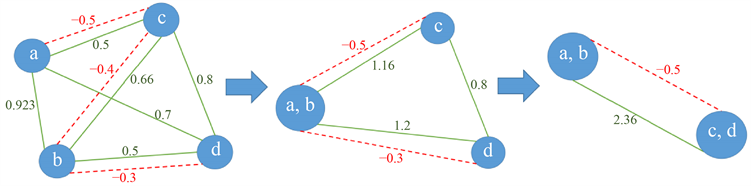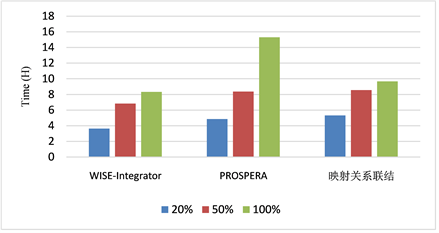﻿ 基于映射关系的领域词典抽取算法

# 基于映射关系的领域词典抽取算法A Domain Dictionary Extraction Algorithm Based on Mapping Relationships

Abstract: The domain dictionary is a form of expression of domain knowledge and the important reference information for data normalization and data cleaning. The mapping relationships refer to the cor-responding relationship between two columns in a table. The construction and expansion of domain dictionary takes Web tables as the main data source, and it is necessary to connect and expand the local mapping relationships in many Web tables. However, there are heterogeneous and data qual-ity problems in Web tables, data integration technologies, for example, pattern matching cannot be relied on. This paper proposes a domain dictionary extraction algorithm based on mapping rela-tions. Firstly, we use the IDF-Jaccard maximum containment and edit distance for approximate string matching, and use Gaussian mixture model to achieve numerical discretization, thereby solving the heterogeneity problem at the data level. Next, the candidate table containing mapping relationships is determined by the pointwise mutual information and functional dependence; then the compatibility and repulsion between the candidate tables are defined, and the mapping rela-tionship graph model is constructed to connect the candidate tables, and the domain dictionary with the form of mapping relationships is extracted. Finally, to ensure the quality of the domain dic-tionary, a conflict resolution process was added. In the experimental verification, this paper used real estate data sets, compared with other algorithms that obtain domain knowledge from the Web, so the effectiveness and reliability of the algorithm proposed was verified.

1. 引言Table 1. A table of school district allocationTable 2. A table of real estate informationTable 4. A table of block informationTable 5. A table of supporting facility

2. 相关工作

3. 短文本近似字符串匹配算法

3.1. 带IDF权重的Jaccard最大包含度

Jaccard包含度(Jaccard Containment) ，表示为JCont，其公式为

$\text{JCont}\left({\text{Str}}_{1},{\text{Str}}_{2}\right)=\frac{|{\text{Str}}_{1}\cap {\text{Str}}_{2}|}{|{\text{Str}}_{1}|}$ (1)

${\text{JCont}}_{\text{IDF}}\left({\text{Str}}_{1},{\text{Str}}_{2}\right)=\frac{{\text{weight}}_{\text{IDF}}\left({\text{Str}}_{1}\cap {\text{Str}}_{2}\right)}{{\text{weight}}_{\text{IDF}}\left({\text{Str}}_{1}\right)}$ (2)

$\text{Str}=\left\{{a}_{1},{a}_{2},{a}_{3},\cdots ,{a}_{n}\right\}$${\text{weight}}_{\text{IDF}}\left(\text{Str}\right)={\sum }_{1}^{n}\text{IDF}\left({a}_{n}\right)$ ，其中 $\text{IDF}\left({a}_{n}\right)=\mathrm{log}\frac{|{A}_{\text{all}}|}{|\left\{{A}_{m}:{a}_{n}\in {A}_{m}\right\}|+1}$$|{A}_{\text{all}}|$ 指表格

${\text{MJCont}}_{\text{IDF}}\left({\text{Str}}_{1},{\text{Str}}_{2}\right)=\mathrm{max}\left\{\frac{{\text{weight}}_{\text{IDF}}\left({\text{Str}}_{1}\cap {\text{Str}}_{2}\right)}{{\text{weight}}_{\text{IDF}}\left({\text{Str}}_{1}\right)},\frac{{\text{weight}}_{\text{IDF}}\left({\text{Str}}_{1}\cap {\text{Str}}_{2}\right)}{{\text{weight}}_{\text{IDF}}\left({\text{Str}}_{2}\right)}\right\}$ (3)

3.2. 相对编辑距离阈值

${\theta }_{\text{ed}}=\mathrm{min}\left\{⌊|{\text{Str}}_{1}|\cdot {f}_{\text{ed}}⌋,⌊|{\text{Str}}_{2}|\cdot {f}_{\text{ed}}⌋,{k}_{\text{ed}}\right\}$ (4)

3.3. 近似字符串匹配

4. 基于高斯混合模型的离散化算法

$P\left(y|\theta \right)=\underset{k=1}{\overset{K}{\sum }}{\alpha }_{k}\varphi \left(y|{\theta }_{k}\right)$ (5)Figure 1. Preliminary division of intervals based on GMM

${\text{CT}}_{k}=\frac{{N}_{k}}{{N}_{\text{All}}}\ast {\alpha }_{k}$ (6)

${\mathrm{upper}{r}^{\prime }}_{k}={\text{upper}}_{k}+\frac{{\text{CT}}_{k}}{{\text{CT}}_{k}+{\text{CT}}_{k+1}}{\text{lower}}_{k+1}-{\text{upper}}_{k}$ (7)

${\mathrm{lowe}{r}^{\prime }}_{k+1}={\text{lower}}_{k+1}-\frac{{\text{CT}}_{k+1}}{{\text{CT}}_{k}+{\text{CT}}_{k+1}}{\text{lower}}_{k+1}-{\text{upper}}_{k}$ (8)

5. 基于映射关系联结的领域知识抽取算法

5.1. 相关概念

5.2. 映射关系提取

5.2.1. 基于PMI (点互信息)的列过滤

$s\left(u,v\right)$ 为值u和值v间的语义相似性， $\mathcal{C}\left(u\right)=\left\{C|u\in C,C\in T,T\in \mathcal{T}\right\}$ 表示在Web表格语料库 $\mathcal{T}$ 中包含值u的列集，同样 $\mathcal{C}\left(v\right)=\left\{C|v\in C,C\in T,T\in \mathcal{T}\right\}$ 指Web表格语料库 $\mathcal{T}$ 中包含值v的列集。如果集合 $\mathcal{C}\left(u\right)\cap \mathcal{C}\left(v\right)$ 的基数较大，就意味着u和v会频繁地共同出现(例如，当u为辽宁，v为黑龙江时)。它们间具有较高的语义相似性。

$\text{PMI}\left(u,v\right)=\mathrm{log}\frac{p\left(u,v\right)}{p\left(u\right)p\left(v\right)}$ (9)

$s\left(u,v\right)=\text{NPMI}\left(u,v\right)=\frac{\text{PMI}\left(u,v\right)}{-\mathrm{log}p\left(u,v\right)}$ (10)

$\stackrel{¯}{C}=\frac{{\sum }_{{v}_{i},{v}_{j}\in C,i (11)

5.2.2. 基于函数依赖的候选表过滤

5.3. 关系图模型下的映射关系联结

5.3.1. 映射关系间的相容性

${J}^{+}\left(B,{B}^{\prime }\right)=\mathrm{max}\left\{\frac{|B\cap {B}^{\prime }|}{|B|},\frac{|B\cap {B}^{\prime }|}{|{B}^{\prime }|}\right\}$ (12)

$P\left(m|{n}_{a},{n}_{b},{n}_{D}\right)=\frac{{C}_{{n}_{a}}^{S}\cdot {C}_{{n}_{D}-{n}_{a}}^{{n}_{b}-m}}{{C}_{{n}_{D}}^{{n}_{b}}}$ (13)

${C}_{h}^{+}\left(B,{B}^{\prime }\right)=F\left(t|B,{B}^{\prime }\right)=\underset{m\in \left[0,t\right]}{\sum }\text{ }\text{ }P\left(m|{n}_{a},{n}_{b},{n}_{D}\right)$ (14)

${C}^{+}\left(B,{B}^{\prime }\right)=\mathrm{max}\left\{{J}^{+}\left(B,{B}^{\prime }\right),{C}_{h}^{+}\left(B,{B}^{\prime }\right)\right\}$ (15)

5.3.2. 映射关系间的相斥性

${R}^{-}\left(B,{B}^{\prime }\right)=-\mathrm{max}\left\{\frac{|\text{Conflict}\left(B,{B}^{\prime }\right)|}{|B|},\frac{|\text{Conflict}\left({B}^{\prime },B\right)|}{|{B}^{\prime }|}\right\}$ (16)

5.3.3. 映射关系联结Figure 2. A diagram of mapping relationships synthesizing

$\mathcal{B}$ 划分为不相交的分区(子图)的原则是：1) 将相容表分到一起，以尽可能地增加单个映射关系的覆盖范围；2) 相斥表不应当放到同一个分区(子图)中。具体来说：

${R}^{-}\left(P\right)={\sum }_{{B}_{i},{B}_{j}\in P,{R}^{-}\left({B}_{i},{B}_{j}\right)<\tau }{R}^{-}\left({B}_{i},{B}_{j}\right)$ ，所有分区(子图)中应满足约束 ${\sum }_{P\in \mathcal{P}}{R}^{-}\left(P\right)=0$

5.4. 冲突消解

$\begin{array}{l}\text{maximize}\text{\hspace{0.17em}}|\underset{{B}_{i}\in {P}_{T}}{\cup }{B}_{i}|\\ \text{subjectto}\text{\hspace{0.17em}}\text{ }\text{Conflict}\left({B}_{i},{B}_{j}\right)=\varnothing ,\text{\hspace{0.17em}}\text{\hspace{0.17em}}\forall {B}_{i},{B}_{j}\in {P}_{T}\end{array}$ (17)

6. 实验

6.1. 数据集

6.2. 评价指标

$\text{f}{1}_{\text{score}}=2\text{precision}\ast \text{recall}/\text{precision}+\text{recall}$

6.3. 实验结果Table 6. The comparison of experimental resultsFigure 3. Run time comparison

7. 总结

 王鑫, 邹磊, 王朝坤, 彭鹏, 冯志勇. 知识图谱数据管理研究综述[J]. 软件学报, 2019, 30(7): 2139-2174. http://www.jos.org.cn/1000-9825/5841.htm

 Abadi, D.J., Marcus, A. and Madden, S.R. (2009) SW-Store: A Ver-tically Partitioned DBMS for Semantic Web Data Management. VLDB Journal, 18, 385-406.
https://doi.org/10.1007/s00778-008-0125-y

 Abadi, D.J., Marcus, A. and Madden, S.R. (2007) Scalable Se-mantic Web Data Management Using Vertical Partitioning. In: Klas, W., Ed., Proceedings of the 33rd International Con-ference on Very Large Data Bases, VLDB Endowment, Vienna, 411-422.

 陈文亮, 朱靖波, 朱慕华, 姚天顺. 基于领域词典的文本特征表示[J]. 计算机研究与发展, 2005, 42(12): 2155-2160.

 宋施恩, 樊兴华. 基于词共现和词上下文的领域观点词抽取方法[J]. 计算机工程与设计, 2013, 34(11): 4012-4015.

 Venetis, P., Halevy, A.Y., Madhavan, J., Pasca, M., Shen, W., Wu, F., Miao, G.X. and Wu, C. (2011) Recovering Semantics of Tables on the Web. PVLDB, 4, 528-538.
https://doi.org/10.14778/2002938.2002939

 Hearst, M.A. (1992) Automatic Acquisition of Hyponyms from Large Text Corpora. Proceedings of the 14th Conference on Computational Linguistics, Volume 2, 539-545.
https://doi.org/10.3115/992133.992154

 Banko, M., Cafarella, M.J., Soderland, S., Broadhead, M. and Etzioni, O. (2007) Open Information Extraction from the Web. Proceedings of IJCAI, Volume 7, 2670-2676.

 Lehmberg, O. and Bizer, C. (2017) Stitching Web Tables for Improving Matching Quality. PVLDB, 10, 1502-1513.
https://doi.org/10.14778/3137628.3137657

 Ling, X., Halevy, A.Y., Wu, F. and Yu, C. (2013) Synthesizing Union Tables from the Web. Proceedings of the Twenty-Third International Joint Conference on Artificial Intelligence, Beijing, 3-9 August 2013, 2677.

 Kang, J. and Naughton, J.F. (2003) On Schema Matching with Opaque Column Names and Data Values. SIGMOD 2003, San Diego, 9-12 June 2003, 205-216.
https://doi.org/10.1145/872757.872783

 Nargesian, F., Zhu, E.K., Pu, K.Q. and Miller, R.J. (2018) Table Un-ion Search on Open Data. Proceedings of the VLDB Endowment, 11, 813-825.
https://doi.org/10.14778/3192965.3192973

 Chaudhuri, S., Ganti, V. and Kaushik, R. (2006) A Primitive Op-erator for Similarity Joins in Data Cleaning. 22nd International Conference on Data Engineering (ICDE’06), Atlanta, 3-7 April 2006, 5.
https://doi.org/10.1109/ICDE.2006.9

 Agrawal, P., Arasu, A. and Kanshik, R. (2010) On Indexing Er-ror-Tolerant Set Containment. In: Proceedings of the 2010 International Conference on Management of Data, ACM Press, Indianapolis, 927-938.
https://doi.org/10.1145/1807167.1807267

 Ukkonen, E. (1985) Algorithms for Approximate String Matching. Information and Control, 64, 100-118.
https://doi.org/10.1016/S0019-9958(85)80046-2

 Khanmohammadi, S. and Chou, C.-A. (2016) A Gaussian Mixture Model Based Discretization Algorithm for Associative Classification of Medical Data. Expert Systems with Ap-plications, 58, 119-129.
https://doi.org/10.1016/j.eswa.2016.03.046

 Bouma, G. (2009) Normalized (Pointwise) Mutual Information in Collocation Extraction. Proceedings of GSCL, Potsdam, September 2009, 31-40.

 Miller, R.J., Nargesian, F., Christodoulakis, C., Pu, K.Q. and Andritsos, P. (2018) Making Open Data Transparent: Data Discovery on Open Data. IEEE Data Engineering Bulletin, 41, 59-70.

 Wang, Y. and He, Y. (2017) Synthesizing Mapping Relationships Us-ing Table Corpus. Proceedings of the 2017 ACM International Conference on Management of Data, SIGMOD Confer-ence 2017, Chicago, 14-19 May 2017.
https://doi.org/10.1145/3035918.3064010

 Nakashole, N., Theobald, M. and Weikum, G. (2011) Scalable Knowledge Harvesting with High Precision and High Recall. Conference on Web Search and Data Mining (WSDM 2011), Hong Kong, 9-12 February 2011, 227-236.
https://doi.org/10.1145/1935826.1935869

 He, H., Meng, W., Yu, C.T. and Wu, Z. (2003) WISE-Integrator: An Automatic Integrator of Web Search Interfaces for E-Commerce. Proceedings 2003 VLDB Conference, Berlin, 9-12 September 2003, 357-368.
https://doi.org/10.1016/B978-012722442-8/50039-2

Top